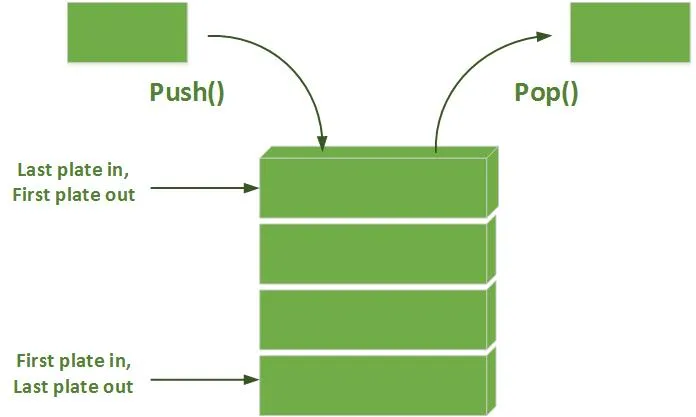# Programming - C Stacks using Linked ListsAfter talking about Queues, we will also talk about Stacks using Linked Lists. We will implement the same functions we did when using Arrays (push, pop, print). So, without further do, let's get started!

As we already know, a Stack is a Data Structure that implements the LIFO way of getting information. We define the most Data Structures with structs in C. But, because this time we use Lists we will also need a Node for the List. This Node will contain Data and a pointer to the next Node. So our Node looks like this:

``typedef struct Node{ ``
`` int val;``
`` struct Node *next;``
``}Node;``

Using this Node definition we can now have our Stack top pointer be a Node pointer! So, our Stack struct will contain this pointer and maybe even other informations like capacity of Stack and so on...
The Code looks like this:

``typedef struct Stack{``
`` Node *top;``
``}Stack;``

The top pointer will be initialized as NULL, that means the Stack is Empty!

The functions that use a Stack will have an struct variable of type Stack and we will use this variable for doing stuff like adding, removing an Item or printing the Stack. The adding and removing need the address so we have a stack pointer, and for printing we will use a variable so that we don't lose the Stack informations.

For adding we can check if stack is full that means we can't add a new Item, when having a capacity variable. Afterwards, we have to create a new Node the same we we did it in Linked Lists like this:

``Node *nn;``
``// create new node``
``nn = (Node*)malloc(sizeof(Node));``
``nn->val = val;``
``nn->next = NULL;``

A special Case is when the List or Stack is empty, then we have to set top to be new Node. When there are Items inside we have to make top be the next of the new Node and afterwards make new Node our new Top.

So, our Code looks like this:

``void push(Stack *S, int val){``
`` Node *nn;``
`` // create new node``
`` nn = (Node*)malloc(sizeof(Node));``
`` nn->val = val;``
`` nn->next = NULL; ``
`` // check if items inside``
`` if(S->top != NULL){``
``     // make nn's next point to top``
``     nn->next = S->top;``
`` }   ``
`` // set nn to new top``
`` S->top = nn;     ``
``}``

# Removing (Pop):

When removing an item we have to check if stack is empty(that means we return -1), store the value in top index in a temporary variable, make top equal to its next pointer and return the value.

So, our Code looks like this:

``int pop(Stack *S){``
`` int val;``
`` // check if empty``
`` if(S->top == NULL){``
``     return -1;``
`` }``
`` // get value in top``
`` val = S->top->val;``
`` // make top point to next``
`` S->top = S->top->next;``
`` //return the value ``
`` return val; ``
``}``

# Printing:

Last but not least comes printing. We will pass the variable and not the address and call the pop function as long as there are items.

The Code looks like this:

``void print(Stack S){``
`` int val;``
`` // check if empty``
`` if(S.top == NULL){``
``     printf("Stack is Empty!\n");``
``     return;``
`` }``
`` // call pop as long as there are items``
`` while((val = pop(&S)) != -1){``
``     printf("%d ", val);``
`` }   ``
`` printf("\n");``
``}``

# Code:

Last for today here a Code that uses those functions so that you know how to set a Stack up and use it. It's the exact same Code that we did using Arrays. The only thing that changes is the initialization.

``int main(){``
`` //initialize Stack``
`` Stack S;``
`` S.top = NULL;``
`` print(S);``
`` // add 3 to stack``
`` push(&S, 5);``
`` print(S);``
`` // add 7 to stack``
`` push(&S, 10);``
`` print(S);``
`` // add 2 to stack``
`` push(&S, 2);``
`` print(S);``
`` // remove from stack``
`` int val;``
`` val = pop(&S);``
`` if(val == -1){``
``     printf("Queue is Empty!\n");``
`` }``
`` else{``
``     printf("%d\n", val);``
`` }``
`` print(S);   ``
``}``

That was the end of this Post. Hope you enjoyed it! :)

Tomorrow I will upload the Solution for the Java Exercise.

H2
H3
H4
3 columns
2 columns
1 column
Join the conversation now